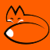Mathias FuchsBlogs Tweener Academic papers CV Past Projects Selected Showcases Home
Inferential statistics of stress states in rigid body continuum mechanics Series of four finite element calculations of a 2d rectangular element under shear stress, subject to random perturbations in each iteration, with first and second principal stress lines in blue and orange (deformations not to scale). In this project, we develop a framework for statistical analysis of stress fluctuations as in this series. In each subfigure, the point at the centre is associated with a particular two-by-two Cauchy stress tensor. A component-wise averaging procedure, resulting in the two-by-two mean Cauchy stress is not an adequate averaging procedure because it disrespects the magnitudes of its eigenvalues, the principal stresses. However, we propose a methodology for averaging and comparing large numbers of perturbed stress samples, circumventing this problem. We thereby allow the researcher to compute measures for the degree of certainty of difference in means between two sets of samples. An important application is quality control of finite element simulations.
Geometry and shell structures Rhino3d grasshopper plugin that designs membranes in real-time and computes approximate solutions to the classical Poisson problem of PDE theory, using a complex analysis-based algorithm instead of a direct matrix method.
A spatial process interpretation of pedestrian indoor tracks learn simulated and observed pedestrian flows as spatial processes, and extrapolating them in real-time to new geometries.
Predicting pedestrian density flow - Real-time occupation modelling in Rhino
A spatial visualisation of pedestrian tracks and density
Real-time prediction of tracks
Non-stationary heat diffusion
Computational fluid dynamics - port of classical code by Stam, Taxen, Nealen to modern fftw3
A simple shape recognition of a 2D office layout by the straight skeleton
Wrapping mmgtool for Rhino3d